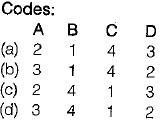Courses

# Test: Basic Concept - 2

## 10 Questions MCQ Test Thermodynamics | Test: Basic Concept - 2

Description
This mock test of Test: Basic Concept - 2 for Mechanical Engineering helps you for every Mechanical Engineering entrance exam. This contains 10 Multiple Choice Questions for Mechanical Engineering Test: Basic Concept - 2 (mcq) to study with solutions a complete question bank. The solved questions answers in this Test: Basic Concept - 2 quiz give you a good mix of easy questions and tough questions. Mechanical Engineering students definitely take this Test: Basic Concept - 2 exercise for a better result in the exam. You can find other Test: Basic Concept - 2 extra questions, long questions & short questions for Mechanical Engineering on EduRev as well by searching above.
QUESTION: 1

Solution:
QUESTION: 2

Solution:
QUESTION: 3

### Match List-I with List-I I and select the correct answer: List-I A. Interchange of matter is not possible in a B. Any processes in which the system returns to its original condition or state is called C. Interchange of matter is possible in a D. The quantity of matter under consideration in thermodynamics is called List-ll 1. Open system 2. System 3. Closed system 4. CycleSolution:
QUESTION: 4

Which one of the following represents open thermodynamic system?

Solution:
QUESTION: 5

A thermodynamic system is considered to be an isolated one if

Solution:
QUESTION: 6

A control volume is

Solution:
QUESTION: 7

Athermodynamic system refers to

Solution:

Explanation : A thermodynamic system is a quantity of matter of fixed identity, around which we can draw a boundary The boundaries may be fixed or moveable. Work or heat can be transferred across the system boundary. Everything outside the boundary is the surroundings.

When working with devices such as engines it is often useful to define the system to be an identifiable volume with flow in and out. This is termed a control volume.

A closed system is a special class of system with boundaries that matter cannot cross. Hence the principle of the conservation of mass is automatically satisfied whenever we employ a closed system analysis. This type of system is sometimes termed a control mass.

QUESTION: 8

In highly rarefied gases, the concept of this loses validity

Solution:

The concept of continuum loses validity when the mean free path of the molecules approaches the order of magnitude of the dimension of the vessel. So, in highly rarefied gases the concept of continuum loses its validity.

QUESTION: 9

Which of the following is an example of heterogeneous system?

Solution:
QUESTION: 10

Consider the following:
1. Temperature
2. Viscosity
3. Specific entropy
4. Thermal conductivity

Which of the above properties of a system is/are intensive

Solution: